Courses

# Test: Concentration, Empirical & Molecular Formulae

## 17 Questions MCQ Test Chemistry for JEE | Test: Concentration, Empirical & Molecular Formulae

Description
This mock test of Test: Concentration, Empirical & Molecular Formulae for JEE helps you for every JEE entrance exam. This contains 17 Multiple Choice Questions for JEE Test: Concentration, Empirical & Molecular Formulae (mcq) to study with solutions a complete question bank. The solved questions answers in this Test: Concentration, Empirical & Molecular Formulae quiz give you a good mix of easy questions and tough questions. JEE students definitely take this Test: Concentration, Empirical & Molecular Formulae exercise for a better result in the exam. You can find other Test: Concentration, Empirical & Molecular Formulae extra questions, long questions & short questions for JEE on EduRev as well by searching above.
QUESTION: 1

### For the reaction 2x + 3y + 4z → 5w Initially if 1 mole of x, 3 mole of y and 4 mole of z is taken. If 1.25 mole of w is obtained then % yield of this reaction is

Solution:

1 moles of x will give = 5/2 = 2.5 mol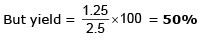QUESTION: 2

### Molarity of NaOH in a solution prepared by dissolving 4 g of NaOH in enough water to form 250 ml of solution is:​

Solution: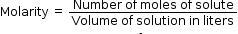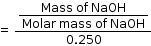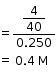QUESTION: 3

### 125 ml of 8% w/w NaOH solution (sp. gravity 1) is added to 125 ml of 10% w/v HCl solution. The nature of resultant solution would be ____

Solution: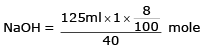= 0.25 mole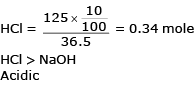QUESTION: 4

Ratio  of masses of H2SO4  and Al2 (SO4)3 is grams each containing 32 grams of S is _____

Solution: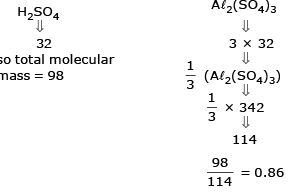QUESTION: 5

18 g of glucose (C6H12O6) is present in 1000 g of an aqueous solution of glucose. The molality of this solution is:​

Solution:

18 g of glucose (C6H12O6) is present in 1000 g of an aqueous solution of glucose. The molality of this solution is 0.1 M.

QUESTION: 6

For the reaction 2A + 3B + 5C → 3D

Initially if 2 mole of A, 4 mole of B and 6 mole of C is taken, With 25% yield, moles of D which can be produced are _____________.

Solution:

Limiting reactant is A
Ideally with 2 moles of A, D formed = 3 moles But yield = 25%
So, moles of D formed = 3 × 0.25 = 0.75 mol

QUESTION: 7

Two elements X (atomic mass = 75) and Y (atomic mass = 16) combine to give a compound having 75.8% of X. The formula of the compound is:

Solution: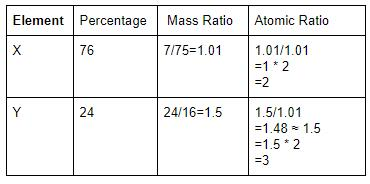So empirical formula of compound = X₂Y₃

For a molecular formula you have to provide molecular mass of the compound.

QUESTION: 8

Equal volumes of 10% (v/v) of HCl is mixed with 10% (v/v) NaOH solution. If density of pure NaOH is 1.5 times that of pure HCl then the resultant solution be :

Solution:

Both have equal volume = V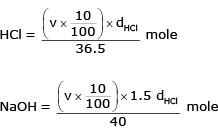NaOH mole > HCl mole
Basic Solution

QUESTION: 9

Similar to the % labelling of oleum, a mixture of H3PO4 and P4O10 is labelled as (100 + x) % where x is the maximum mass of water which can react with P4O10 present in 100 gm mixture of H3PO4 and P4O10. If such a mixture is labelled as 127% Mass of P4O10 is 100 gm of mixture, is

Solution:

P4O10 + 6 H2O → 4H3 PO4
284 gm 108gm 392 gm 108 gm water reacts with P4O10 = 284 gm
27 gm water will react with P4O10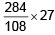= 71 gm

QUESTION: 10

C6H5OH(g) + O2(g) → CO2(g) + H2O(l)

Magnitude of volume change if 30 ml of C6H5OH (g) is burnt with excess amount of oxygen, is

Solution:

C6H5OH(g)+7O2(g) → 6CO2(g)+3H2O(l)
30 ml
6 x 30= 180 ml of CO2 is produced
Volume used initially  = 30 + 210 = 240
(for C6H5OH)(For O2)
change in volume = 240 - 180 = 60 ml

QUESTION: 11

How many moles of NaCl should be dissolved in 100 mL of water to get 0.2 M solution?​

Solution:

Molarity = no of moles /volume( in litre) 0.2 =no of moles / 0.1 No of moles =0.2 × 0.1 = 0.02

QUESTION: 12

If 50 gm oleum sample rated as 118% is mixed with 18 gm water, then the correct option is

Solution:

118 % ⇒ 100 g sample uses 18 g water
⇒ 50 g sample need 9 g water
(50 g + 18 g water)
⇒ 59 g H2SO4 + 9 g water

QUESTION: 13

The minimum mass of mixture of A2 and B4 required to produce at least 1 kg of each product is:
(Given At. mass of ‘A’ = 10; At mass of ‘B’ = 120) 5A2 + 2B4 → 2AB2  + 4A2B

Solution: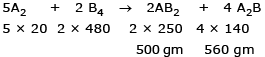Here limiting product is AB2 = 500 gm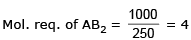So, A2 needed = 10 × 20 = 200 gm
B2 needed = 480 × 4 = 1920 gm
Total mass of mixture = 2120 gm

QUESTION: 14

The % by volume of C4H10 in a gaseous mixture of C4H10, CH4 and CO is 40. When 200 ml of the mixture is burnt in excess of O2. Find volume (in ml) of CO2 produced.

Solution:

C4H10 = 80 ml
CH4 = xml  CO = y ml
x + y = 120 ml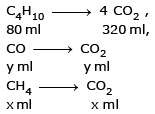total CO2 volume
= 320 + x + y ml
= 320 + 120
= 440 ml

QUESTION: 15

74 gm of sample on complete combustion gives 132 gm CO2 and 54 gm of H2O. The molecular formula of the compound may be

Solution: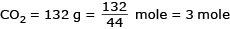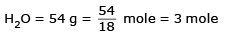⇒ C atoms = 3 mole
H atoms = 6 mole

QUESTION: 16

Density of a gas relative to air is 1.17. Find the mol. mass of the gas. [Mair = 29 g/mol]

Solution: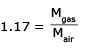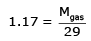M gas = 29 × 1.17 = 33.9

QUESTION: 17

Number of moles of hydroxide ions in 0.3L of 0.005M solution of Ba (OH)2 is:

Solution:

0.3 L = 0.3 L of 0.005 M Ba(OH)2
0.3 x 0.005 = 0.0015 moles of Ba(OH)2
It will dissociate into 0.0015 moles of Ba and 2 x 0.0015 = 0.003 moles of OH ions.